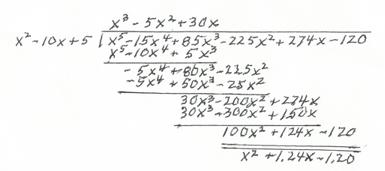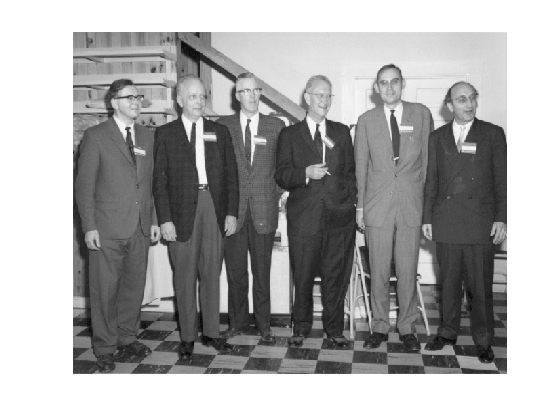# Reduced Penultimate Remainder

I investigated the reduced penultimate remainder algorithm in an undergraduate research project under professor John Todd at Caltech in 1961. I remember it today for two reasons. First, I learned what penultimate means. And second, it is the most obscure, impractical algorithm that I know. I suspect none of my readers have ever heard of it.

### Contents

#### Penultimate

Penultimate means "next to last". November is the penultimate month of the year. In the fifty-plus years since I learned its meaning, I've never had a chance to use penultimate in everyday conversation, or in my writing. I think of it as British English. Is it?

#### Reduced Penultimate Remainder

Start with two polynomials, both with leading coefficient equal to one. When you divide one polynomial into the other using long division, you usually stop when the degree of the remainder is one less than the degree of the divisor. But instead, stop one step earlier, with the penultimate remainder. Then scale the leading coefficient of the remainder to be equal to one. This is the reduced penultimate remainder. If the original divisor had actually been an exact factor of the dividend, then this reduced remainder would be equal to the divisor. Iterating the process with exact divisors exhibits fixed points.

#### An Example

Our example involves dividing $q(x) = x^2 - 10x + 5$ into $p(x) = x^5 - 15x^4 + 85x^3 - 225x^2 + 274x - 120$. Here is a scan of one step of the long division carried out by hand. (I don't know enough LaTeX to be able to do the typesetting.)The reduced penultimate remainder is $r(x) = x^2 + 1.24x - 1.20$. Since $r(x)$ is not close to $q(x)$, we can conclude that $q(x)$ is not close to a factor of $p(x)$ and consequently that the roots of $q(x)$ are not close to the roots of $p(x)$. I will return to this example later.

#### Reduced Penultimate Remainder Algorithm

If the divisor happens to be a factor of the dividend, then the reduced penultimate remainder process is a fixed point iteration. In 1943, Shi-nge Lin suggested that starting with an initial approximation that is nearly a factor and repeating the RPR process, the iteration might converge to a factor and hence could be used to find roots of polynomials. I do not know who Shi-nge Lin was and have not actually seen his original paper.

#### A. C. Aitken

A. C. Aitken(1895-1967) wrote papers about Lin's idea in 1952 and 1955. Aitken was born in New Zealand, educated in Scotland, and became a professor of mathematics at the University of Edinburgh. He made many contributions to many areas of mathematics. In numerical analysis he is best known for the delta-squared sequence acceleration technique. His prodigious memory made some aspects of his life unpleasant. He served with the New Zealand forces in World War I and his memories of the battle at Gallipoli haunted him throughout his life.

John Todd was the professor at Caltech who introduced me to numerical analysis, matrices, and computing. I believe that Todd knew Aitken personally and he was certainly familiar with his work. So he suggested I investigate the method and I read Aitken's papers. I wrote programs in machine language for the Burroughs 205 Datatron. Unfortunately, about all I can remember about the project is the name of the algorithm.

Alston Householder(1904-1993) is one of my heroes. I knew him quite well. He's the fourth guy from the left in our photo of the organizing committee of the 1964 Gatlinburg Conference on Numerical Algebra

load gatlin
image(X)
colormap(map)
axis offI just learned that Householder wrote a paper about the Reduced Penultimate Remainder algorithm in 1970, ten years after I did my undergraduate research project. I didn't know about that paper until I came across it while writing this blog.

Householder makes some very candid comments about the earlier papers by Lin and Aitken. He says "Lin's development is complicated and unsuggestive". He says "Aitken's derivation of the conditions for convergence is very sketchy and leaves much to the reader." Householder's paper was published in the SIAM Journal of Applied Math in 1970. I don't think we could make comments like these in SIAM papers today.

Aitken had introduced a device called the catalytic multiplier and Householder tries to explain it. I do not understand his paper at all. Near the end of the paper he says "In notation elsewhere used by the author ..." That is not very helpful. I'm afraid that is this particular paper Alston is no clearer than the authors he is criticizing. I can say this in a blog.

#### MATLAB Code

type rpr

function q = rpr(p,q)
% RPR Reduced Penultimate Remainder.
% r = rpr(p,q), for polynomials p and q.
% Assume p(1) = 1, q(1) = 1.
for c = 1:15
% Polynomial long division.
% Stop when degree of r = degree of q.
n = length(p);
m = length(q);
r = p(1:m);
for k = m+1:n
r = r - r(1)*q;
r = [r(2:end) p(k)];
end
q = r/r(1);
disp(q)
end



#### Example, continued

The example I used in hand calculation above is the polynomial whose roots are the integers from one to five.

p = charpoly(diag(1:5))

p =
1   -15    85  -225   274  -120


The starting guess is a deliberately bad approximation to a quadratic factor.

q = [1 -10 5]

q =
1   -10     5


Let's run 15 iterations

format long
format compact
rpr(p,q)

   1.000000000000000   1.240000000000000  -1.200000000000000
1.000000000000000  -1.067114979620489   0.318854992571954
1.000000000000000  -1.723550954295960   0.821587108699062
1.000000000000000  -2.062229096576079   1.106543069892922
1.000000000000000  -2.272884387512085   1.294527998591067
1.000000000000000  -2.417143826065064   1.428233837285976
1.000000000000000  -2.522010435111559   1.527880806063684
1.000000000000000  -2.601434375494343   1.604615444108659
1.000000000000000  -2.663426234246922   1.665180563853953
1.000000000000000  -2.712940049546721   1.713920778923779
1.000000000000000  -2.753213571017107   1.753767759646395
1.000000000000000  -2.786455813893868   1.786771702201661
1.000000000000000  -2.814227004785662   1.814408345663866
1.000000000000000  -2.837661124583663   1.837765841878627
1.000000000000000  -2.857602508367706   1.857663278238952
ans =
1.000000000000000  -2.857602508367706   1.857663278238952


It's converging, slowly, to the factor $x^2 - 3x + 2$ with roots $1$ and $2$. It takes about 300 iterations to reach this factor to double precision accuracy.

#### Another Example

One of my favorite polynomials is $p(x) = x^3 - 2x - 5$. It's only real root is near $2.09$. Let's try to find it.

p = [1 0 -2 -5]
q = [1 -2]
rpr(p,q)

p =
1     0    -2    -5
q =
1    -2
1.000000000000000  -2.500000000000000
1.000000000000000  -1.176470588235294
1.000000000000000   8.117977528089890
1.000000000000000  -0.078245352175702
1.000000000000000   2.507676417722349
1.000000000000000  -1.165924862052266
1.000000000000000   7.804948516596162
1.000000000000000  -0.084864830447043
1.000000000000000   2.509035084827172
1.000000000000000  -1.164074683720088
1.000000000000000   7.752777799980693
1.000000000000000  -0.086050279678108
1.000000000000000   2.509290208665588
1.000000000000000  -1.163727809437372
1.000000000000000   7.743083431952472
ans =
1.000000000000000   7.743083431952472


No luck, it's not going to converge. The real root repels any attempt to find it with this iteration.

#### Conclusions

Finding polynomial roots is not a very important task and this algorithm is not very good at it anyway. Its convergence properties are too unreliable. So for me, this is just a quirky memory. If anybody else has heard of the reduced penultimate remainder algorithm, please contribute a comment.

#### References

 A. C. AITKEN, Studies in practical mathematics. VII. On the theory of factorizing polynomials by iterated division, Proc. Roy. Soc. Edinburgh Sec..A, 63 (1952), pp. 326-335.

 A. C. AITKEN, Note on the acceleration of Lin's process of iterated penultimate remainder, Quart. J. Mech. Appl. Math., 8 (1955), pp. 251-258.

 SHI-NGE LIN, A method for finding roots of algebraic equations, J. Math. Phys. Mass. Inst. Tech., 22 (1943), pp. 60-77.

 ALSTON S. HOUSEHOLDER, On the penultimate remainder algorithm and the catalytic multiplier, SIAM J. Appl. Math., 19 (1970), pp. 668-671.

Published with MATLAB® R2012b

|